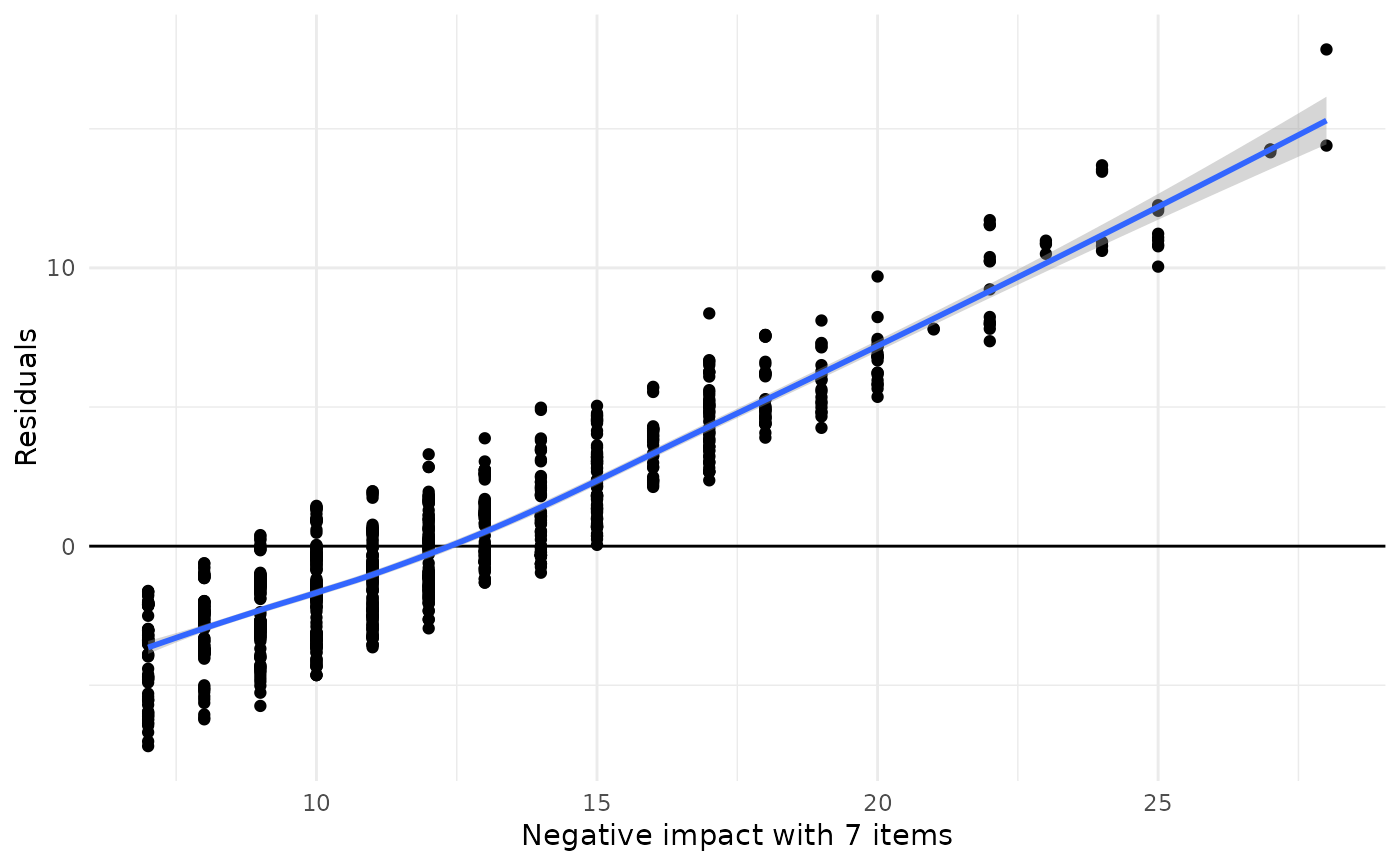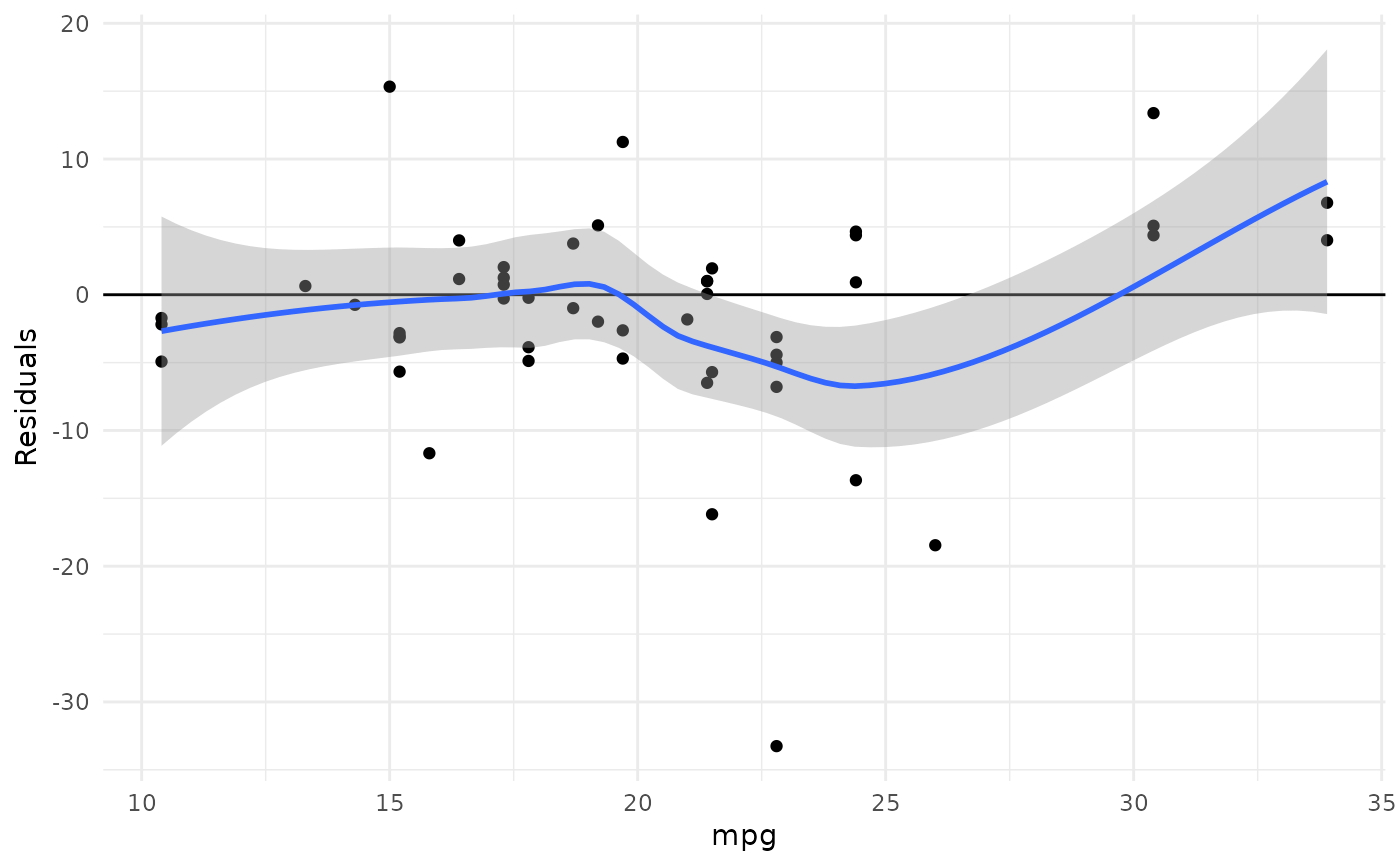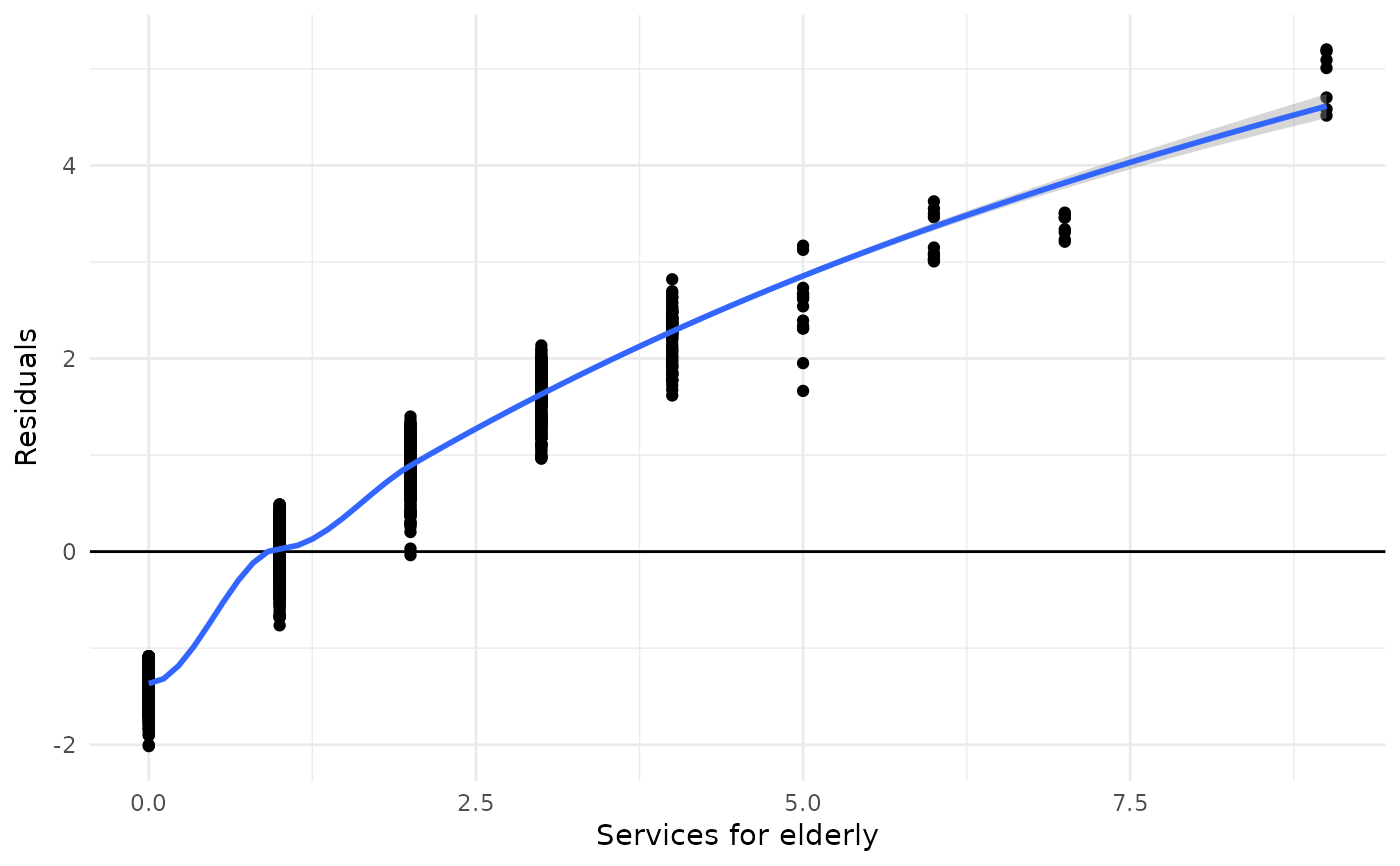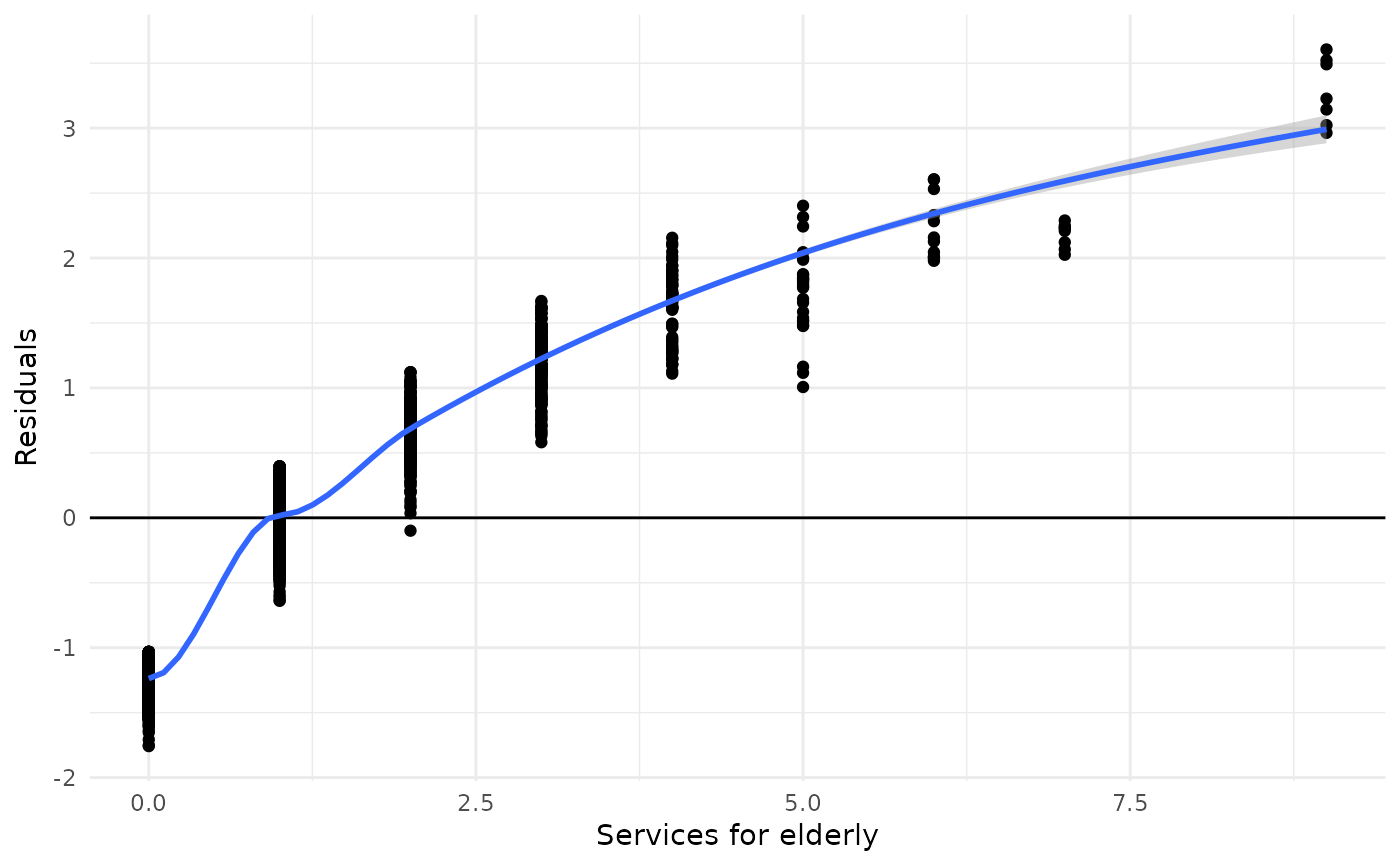This function plots the aggregated residuals of k-fold cross-validated models against the outcome. This allows to evaluate how the model performs according over- or underestimation of the outcome.

plot_kfold_cv(data, formula, k = 5, fit)

Arguments

data

A data frame, used to split the data into k training-test-pairs.

formula

A model formula, used to fit linear models (lm) over all k training data sets. Use fit to specify a fitted model (also other models than linear models), which will be used to compute cross validation. If fit is not missing, formula will be ignored.

k

Number of folds.

fit

Model object, which will be used to compute cross validation. If fit is not missing, formula will be ignored. Currently, only linear, poisson and negative binomial regression models are supported.

Details

This function, first, generates k cross-validated test-training pairs and fits the same model, specified in the formula- or fit- argument, over all training data sets.

Then, the test data is used to predict the outcome from all models that have been fit on the training data, and the residuals from all test data is plotted against the observed values (outcome) from the test data (note: for poisson or negative binomial models, the deviance residuals are calculated). This plot can be used to validate the model and see, whether it over- (residuals > 0) or underestimates (residuals < 0) the model's outcome.

Note

Currently, only linear, poisson and negative binomial regression models are supported.

Examples

data(efc)

plot_kfold_cv(efc, neg_c_7 ~ e42dep + c172code + c12hour)
#> geom_smooth() using formula 'y ~ x'
#> Warning: Removed 66 rows containing non-finite values (stat_smooth).
#> Warning: Removed 66 rows containing missing values (geom_point).plot_kfold_cv(mtcars, mpg ~.)
#> geom_smooth() using formula 'y ~ x'# for poisson models. need to fit a model and use 'fit'-argument
fit <- glm(tot_sc_e ~ neg_c_7 + c172code, data = efc, family = poisson)
plot_kfold_cv(efc, fit = fit)
#> geom_smooth() using formula 'y ~ x'
#> Warning: pseudoinverse used at -0.045
#> Warning: reciprocal condition number  8.8093e-29
#> Warning: There are other near singularities as well. 1
#> Warning: pseudoinverse used at -0.045
#> Warning: reciprocal condition number  8.8093e-29
#> Warning: There are other near singularities as well. 1# and for negative binomial models
fit <- MASS::glm.nb(tot_sc_e ~ neg_c_7 + c172code, data = efc)
plot_kfold_cv(efc, fit = fit)
#> geom_smooth() using formula 'y ~ x'
#> Warning: pseudoinverse used at -0.045